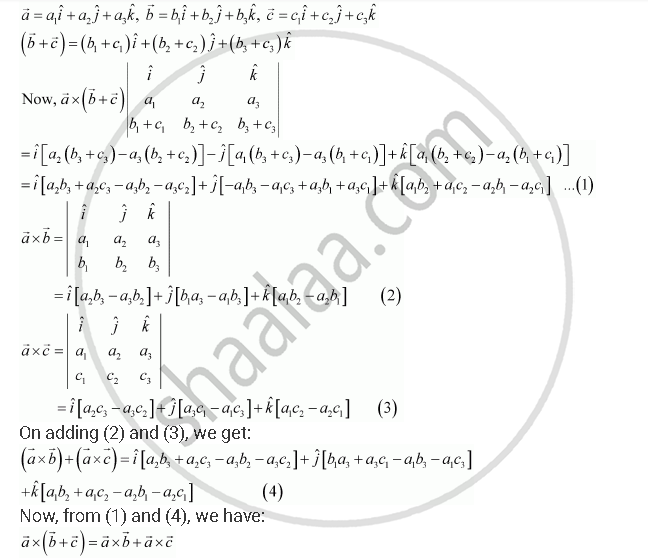Share

# Let the Vectors Veca, Vecb, Vecc Given as A_1hati + A_2hatj + A_3hatk, B_1hati + B_2hatj + B_3hatk, C_1hati + C_2hatj + C_3hatk Then Show that = Veca Xx (Vecb+ Vecc) = Veca Xx Vecb + Veca Xx Vecc - CBSE (Commerce) Class 12 - Mathematics

ConceptProduct of Two Vectors Vector (Or Cross) Product of Two Vectors

#### Question

Let the vectors veca, vecb, vecc given as a_1hati + a_2hatj + a_3hatk, b_1hati + b_2hatj + b_3hatk, c_1hati + c_2hatj + c_3hatk Then show that = veca xx (vecb+ vecc) = veca xx vecb + veca xx vecc

#### Solution

We have,Hence, the given result is proved.

Is there an error in this question or solution?

#### Video TutorialsVIEW ALL 

Solution Let the Vectors Veca, Vecb, Vecc Given as A_1hati + A_2hatj + A_3hatk, B_1hati + B_2hatj + B_3hatk, C_1hati + C_2hatj + C_3hatk Then Show that = Veca Xx (Vecb+ Vecc) = Veca Xx Vecb + Veca Xx Vecc Concept: Product of Two Vectors - Vector (Or Cross) Product of Two Vectors.
S